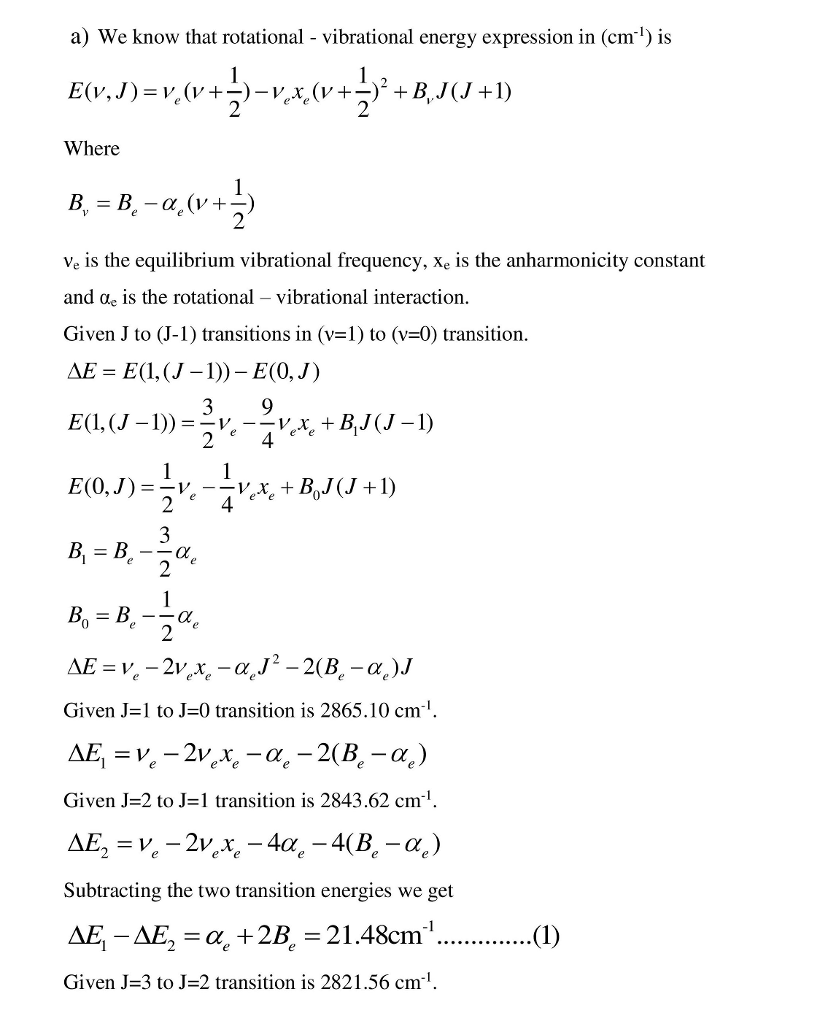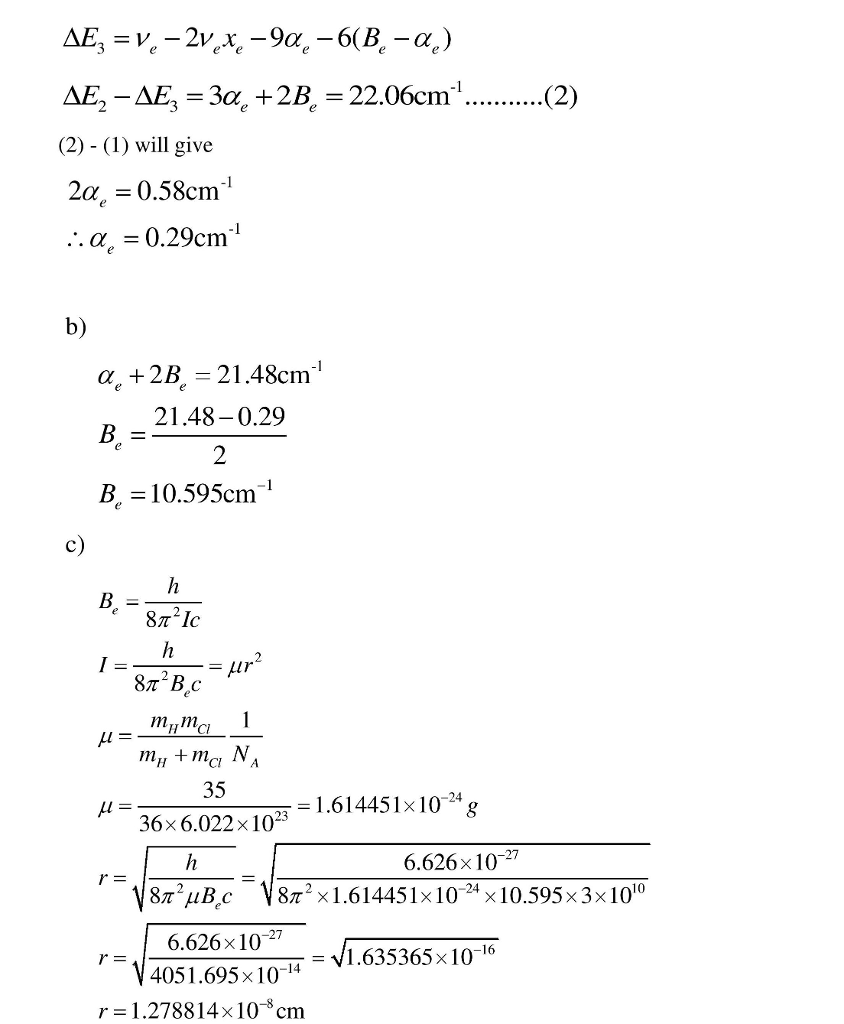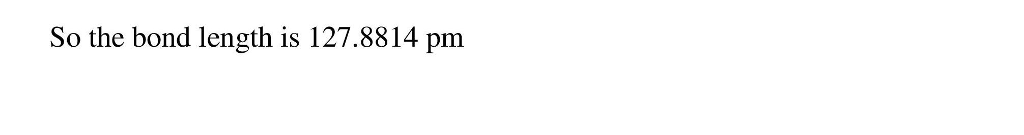In: Chemistry

# The fundamental (v = 0 ! v = 1) vibrational transition for H35Cl occurs at 2885...

The fundamental (v = 0 ! v = 1) vibrational transition for H35Cl occurs at 2885 cm-1 . Close inspection of a high-resolution infrared spectrum of this transition shows the following absorptions (in cm-1 ) for the J ! (J-1) branch: 2865.10, 2843.62, 2821.56, 2798.94, 2775.76, 2752.04, 2727.78, 2703.01, and 2677.73. From these data, determine

a) the rotational-vibrational coupling constant, αe

b) the rotational constant, Be

c) the bond distance

## Solutions

##### Expert Solution## Related Solutions

##### The fundamental vibrational transition of the 12C16O in the electronic ground state from v=0 to v=1...
The fundamental vibrational transition of the 12C16O in the electronic ground state from v=0 to v=1 occurs at 2,170 cm-1. The bottom of the Morse potential for the anharmonic oscillator is well approximated by a parabola, the potential well for the harmonic oscillator, especially when the transition is measured with precision limited to 1 cm-1. a) Demonstrate that, in the harmonic oscillator approximation of the IR spectroscopy, the frequency of the fundamental transition is equal to the frequency of the...
##### Calculate the vibrational energy difference, in cm-1, between the v=0 and v=1 energy level in a...
Calculate the vibrational energy difference, in cm-1, between the v=0 and v=1 energy level in a hydrogen chloride-35 molecule. Isotope masses to three decimal places are taken from NIST and force constants from hyperphysics. Use the isotope mass, not the average mass.
##### a) The J = 0 to J =1 rotational transition of the CO molecule occurs at...
a) The J = 0 to J =1 rotational transition of the CO molecule occurs at a frequency of 1.15×1011 Hz. Use this information to calculate the moment of inertia of the molecule about its center of mass. b) The CO molecule shows a strong absorption line at the frequency 6.42×1013 Hz due to transition from thev= 0 to the v = 1 vibrational levels. Calculate the effective force constant for this molecule. c) Why does CO molecule have pure...
##### The fundamental vibrational frequencies for 1H2and 14N2 are 4401 and 2359 cm−1, respectively, and De for...
The fundamental vibrational frequencies for 1H2and 14N2 are 4401 and 2359 cm−1, respectively, and De for this molecules is 7.677×10−19 and 1.593×10−18 J respectively. Part A Using this information, calculate the bond energy of 1H2. Express your answer to four significant figures and include the appropriate units. EH2 = SubmitMy AnswersGive Up Part B Calculate the bond energy of 14N2. Express your answer to four significant figures and include the appropriate units. EN2 =
##### Bound state in potential. Where V(x)=inf for x<0 V(x)=0 for 0 ≤ x ≤ L V...
Bound state in potential. Where V(x)=inf for x<0 V(x)=0 for 0 ≤ x ≤ L V (x) = U for x > L Write down the Schrödingerequation and solutions (wavesolutions) on general form for the three V(x).
##### 1.Determine whether S spans V . Justify your answers. V = C ^0 [−1, 1] (the...
1.Determine whether S spans V . Justify your answers. V = C ^0 [−1, 1] (the vector space of continuous functions on [−1, 1]) and S = {1, t, t2 , t3 , . . . }. 2.Let S be a set in a vector space V and v any vector. Prove that span(S) = span(S ∪ {v}) if and only if v ∈ span(S).
##### The subject is nursing fundamental 101 at transition Career Institute. The questions on ATI testing ....
The subject is nursing fundamental 101 at transition Career Institute. The questions on ATI testing . and nursing leadership
##### 1-Explain why fundamental attribution error occurs giving four factors and provide an example of this phenomenon...
1-Explain why fundamental attribution error occurs giving four factors and provide an example of this phenomenon that might occur in daily life Four factors are 1- language 2- cultural 3- motivational 4- percetual 2- what is the difference between the fundamental attribution error and the self serving bias ?
##### Solve y'(0)=Ay y(0) =v A=(7,-5;10,-8) v=(2;3)
Solve y'(0)=Ay y(0) =v A=(7,-5;10,-8) v=(2;3)
##### The vibrational wavenumber of Br2 is 323.2 cm-1. a) Evaluate the vibrational partition function without approximation...
The vibrational wavenumber of Br2 is 323.2 cm-1. a) Evaluate the vibrational partition function without approximation and plot its value as a function of temperature. b) Determine the temperature at which the value obtained lies within 5% of the approximate value.